Next: About this document ... Up: lab_template Previous: lab_template

Subsections

# Graphs in Polar Coordinates

## Purpose

The purpose of this lab is to help you become familiar with graphs in polar coordinates.

## Getting Started

To assist you, there is a worksheet associated with this lab that contains examples and even solutions to some of the exercises. You can copy that worksheet to your home directory with the following command, which must be run in a terminal window, not in Maple.

cp /math/calclab/MA1023/Polar_start_C08.mws My_Documents


You can copy the worksheet now, but you should read through the lab before you load it into Maple. Once you have read to the exercises, start up Maple, load the worksheet Polar_start_C08.mws, and go through it carefully. Then you can start working on the exercises.

## Background

There are many times in math, science, and engineering that coordinate systems other than the familiar one of Cartesian coordinates are convenient. In this lab, we consider one of the most common and useful such systems, that of polar coordinates.

The main reason for using polar coordinates is that they can be used to simply describe regions in the plane that would be very difficult to describe using Cartesian coordinates. For example, graphing the circlein Cartesian coordinates requires two functions - one for the upper half and one for the lower half. In polar coordinates, the same circle has the very simple representation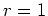.

### Cardioids, Limaçons, and Roses

These are three types of well-known graphs in polar coordinates. The table below will allow you to identify the graphs in the exercises.

 Name Equation cardioid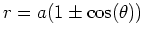or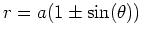limaçonor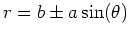roseor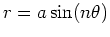### Intersections of Curves in Polar Coordinates

Finding where two graphs in Cartesian coordinates intersect is straightforward. You just set the two functions equal and solve for the values of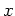. In polar coordinates, the situation is more difficult. Most of the difficulties are due to the following considerations.
1. A point in the plane can have more than one representation in polar coordinates. For example,,is the same point as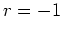,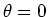. In general a point in the plane can have an infinite number of representations in polar coordinates, just by adding multiples of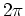to. Even if you restricta point in the plane can have several different representations.
2. The origin is determined by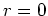. The anglecan have any value.
These considerations can make finding the intersections of two graphs in polar coordinates a difficult task. As the exercises demonstrate, it usually requires a combination of plots and solving equations to find all of the intersections.

## Exercises

1. For each of the following polar equations, plot the graph in polar coordinates using the plot command and identify the graph as a cardioid, limaçon, or rose.
1.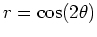2.3.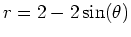4.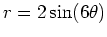2. Find all points of intersection for each pair of curves in polar coordinates.
1.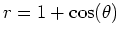and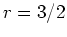for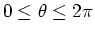.
2.and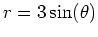for.
3.and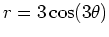for.

3. Using the graph and results from exercise 2, part 3, find the area inside the petal in the second quadrant and outside the cardioid.Next: About this document ... Up: lab_template Previous: lab_template
Dina J. Solitro-Rassias
2009-02-11